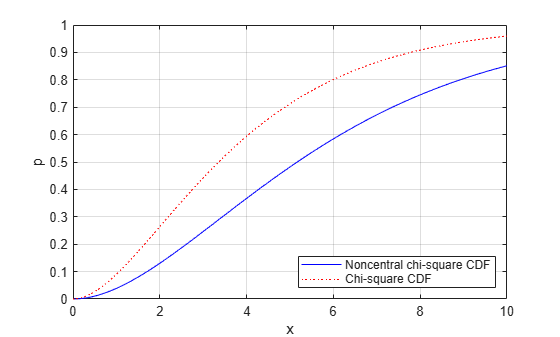Documentation

# ncx2cdf

Noncentral chi-square cumulative distribution function

## Syntax

```p = ncx2cdf(x,v,delta) p = ncx2cdf(x,v,delta,'upper') ```

## Description

`p = ncx2cdf(x,v,delta)` computes the noncentral chi-square cdf at each value in `x` using the corresponding degrees of freedom in `v` and positive noncentrality parameters in `delta`. `x`, `v`, and `delta` can be vectors, matrices, or multidimensional arrays that all have the same size, which is also the size of `p`. A scalar input for `x`, `v`, or `delta` is expanded to a constant array with the same dimensions as the other inputs.

`p = ncx2cdf(x,v,delta,'upper')` returns the complement of the noncentral chi-square cdf at each value in `x`, using an algorithm that more accurately computes the extreme upper tail probabilities.

Some texts refer to this distribution as the generalized Rayleigh, Rayleigh-Rice, or Rice distribution.

The noncentral chi-square cdf is

`$F\left(x|\nu ,\delta \right)=\sum _{j=0}^{\infty }\left(\frac{{\left(\frac{1}{2}\delta \right)}^{j}}{j!}{e}^{\frac{-\delta }{2}}\right)\mathrm{Pr}\left[{\chi }_{\nu +2j}^{2}\le x\right]$`

## Examples

collapse all

Compare the noncentral chi-square cdf with `DELTA = 2` to the chi-square cdf with the same number of degrees of freedom (4):

```x = (0:0.1:10)'; ncx2 = ncx2cdf(x,4,2); chi2 = chi2cdf(x,4); plot(x,ncx2,'b-','LineWidth',2) hold on plot(x,chi2,'g--','LineWidth',2) legend('ncx2','chi2','Location','NW')```## References

 Johnson, N., and S. Kotz. Distributions in Statistics: Continuous Univariate Distributions-2. Hoboken, NJ: John Wiley & Sons, Inc., 1970, pp. 130–148.